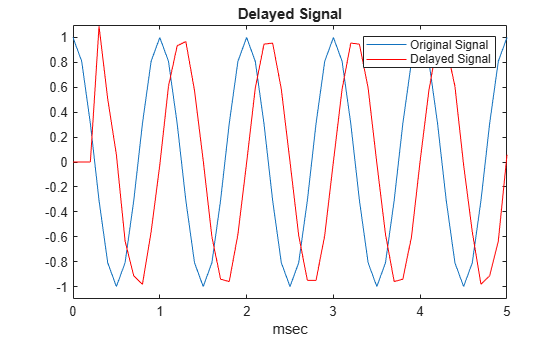# delayseq

## Syntax

``shifted_data = delayseq(data,delay)``
``shifted_data = delayseq(data,delay,fs)``

## Description

example

````shifted_data = delayseq(data,delay)` delays or advances the signal in `data` by the number of samples specified in `delay`. Positive values of `delay` delay the signal, while negative values advance the signal. Noninteger values of `delay` represent fractional delays or advances. For fractional delays, the function interpolates between samples.How the `delayseq` function operates on `data` depends on the dimensions of the `data` and `delay` arguments:When `delay` is a scalar, the function applies the same delay to each column of `data`.When `delay` is a vector:If `data` is a matrix, the length of the `delay` vector must equal the number of columns in the matrix. The function applies a delay to each column using the corresponding `delay` entry.If `data` is a column vector, the function creates a matrix where each column is the shift in the `data` vector by each entry in `delay`. The number of columns in `shifted_data` equals the length of the `delay` vector. The kth column of `shifted_data` is the result of shifting `data` by `delay(k)`.```

example

````shifted_data = delayseq(data,delay,fs)` specifies `delay` in seconds. `fs` is the sampling frequency of `data`. If the product of `delay` and `fs` is not an integer, `delayseq` implements a fractional delay or advance of the signal using interpolation.```

## Examples

collapse all

Delay a 1 kHz cosine signal by an integer number of samples. Assume a sampling rate of 10 kHz.

```fs = 1.0e4; t = 0:1/fs:0.005; signal = cos(2*pi*1000*t)';```

Set the delay to 5 samples (0.5 ms).

`shifted_signal = delayseq(signal,5);`

Plot the original and delayed signals.

```subplot(2,1,1) plot(t.*1000,signal) title('Input') subplot(2,1,2) plot(t.*1000,shifted_signal) title('5 Sample Delay') xlabel('msec')```Delay a 1 kHz cosine signal by a fractional number of samples. Assume a sampling rate of 10 kHz.

```fs = 1e4; t = 0:1/fs:0.005; signal = cos(2*pi*1000*t)';```

Set the delay to 0.25 ms or 2.5 samples.

`delayed_signal = delayseq(signal,0.25e-3,fs);`

Plot the original and delayed signals.

```plot(t.*1000,signal) title('Delayed Signal') hold on plot(t.*1000,delayed_signal,'r') axis([0 5 -1.1 1.1]) xlabel('msec') legend('Original Signal','Delayed Signal') hold off```The delayed signal values differ from the original signal values because interpolation is used to implement the fractional delay.

## Input Arguments

collapse all

Input signal, specified as a real-valued length-M vector, complex-valued length-M vector, real-valued M-by-N matrix, or complex-valued M-by-N matrix.

M is the number of samples in `data`. When `data` is a matrix, N is the number of independent signals.

Data Types: `single` | `double`
Complex Number Support: Yes

Signal delay or advance, specified as a scalar or real-value N-length vector. If you specify the `fs` argument, `delay` units are in seconds. When `delay` is a scalar, the same delay is applied to all columns of `data`. `delay` units are in samples if `fs` is not specified and in seconds if `fs` is specified.

Data Types: `single` | `double`

Sampling frequency of the signal, specified as a positive scalar. Units are in Hz.

Data Types: `single` | `double`

## Output Arguments

collapse all

Delayed or advanced signal, returned as a real-valued length-M vector, complex-valued length-M vector, real-valued M-by-N matrix, or complex-valued M-by-N matrix. `shifted_data` has the same number of rows as `data`, with appropriate truncations or zero padding.

## Version History

Introduced in R2011a